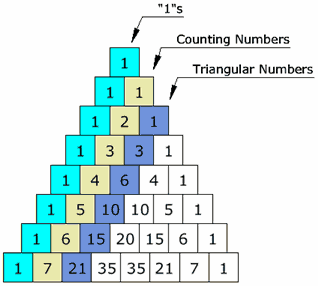# Linear Relationships

##BIG IDEAS:

(taken from “Big Ideas by Dr. Small”):

1. Patterns represent identified regularities. There is always an element of repetition.
2. Patterns can be represented in a variety of ways (i.e. pictures, words, graphs, sequences, tables).
3. Some ways of displaying data highlight patterns.

## STUDENT LEARNING GOALS:

GOAL #1: I can classify, extend and describe patterns.

GOAL #2: I can represent a pattern as a sequence, table of values, picture, graph and in words.

GOAL #3: I can determine the explicit pattern rule (algebraic equation) for a linear pattern.

## CURRICULUM EXPECTATIONS:

• Represent linear growing patterns (where the terms are whole numbers) using concrete materials, graphs, and algebraic expressions;
• Represent linear growing patterns, using a variety of tools (e.g., concrete materials, paper and pencil, calculators, spreadsheets) and strategies (e.g., make a table of values using the term number and the term; plot the coordinates on a graph; write a pattern rule using words);
• Make predictions about linear growing patterns, through investigation with concrete materials;
• Develop and represent the general term of a linear growing pattern, using algebraic expressions involving one operation (e.g., the general term for the sequence 4, 5, 6, 7, … can be written algebraically as n + 3, where n represents the term number; the general term for the sequence 5, 10, 15, 20, … can be written algebraically as 5n, where n represents the term number);
• Compare pattern rules that generate a pattern by adding or subtracting a constant, or multiplying or dividing by a constant, to get the next term (e.g., for 1, 3, 5, 7, 9, …, the pattern rule is “start at 1 and add 2 to each term to get the next term”) with pattern rules that use the term number to describe the general term (e.g., for 1, 3, 5, 7, 9, …, the pattern rule is “double the term number and subtract 1,” which can be written algebraically as 2n – 1).
• Model real-life relationships involving constant rates where the initial condition starts at 0 (e.g., speed, heart rate, billing rate), through investigation using tables of values and graphs;
• Make connections between evaluating algebraic expressions and determining the term in a pattern using the general term (e.g., for 3, 5, 7, 9, …, the general term is the algebraic expression 2n + 1; evaluating this expression when n = 12 tells you that the 12th term is 2(12) + 1, which equals 25);